Superstrings Michael Green Scientific American Sept 86

The central paradox of the contemporary physics of elementary particles is the apparent incompatibility of its two main theoretical foundations. The first foundation is Einstein's general theory of relativity, which relates the force of gravity to the structure of space and time. This view of gravity has led to models of phenomena on a cosmic scale and to an understanding of the evolution of the universe. The second theoretical foundation is quantum mechanics, which can account for the atomic and subatomic world. Quantum theories have been formulated for three of the four known forces of nature: the strong, weak and electromagnetic interactions. Until recently there seemed to be little hope that Einstein's theory of gravity-the fourth fundamental force-could be united with the precepts of quantum mechanics. The basic difficulty is that such a unification seems to call for a radically new formulation of the laws of physics at the smallest distance scales; in such a reformulation the idea that space and time are continuous sets of points would have to be abandoned. Without a quantum theory of gravity and the conceptual revisions such a theory implies, a comprehensive description of all the forces of nature could not be realized. In the past two years elementaryparticle physicists have become optimistic that the theoretical impasse might be resolved. The optimism is based on striking developments in a new kind of theory known as superstring theory. In superstring theory, as in any other string theory, elementary particles can be thought of as strings. String theories thereby differ from all familiar quantum-mechanical field theories, such as the quantum theory of electromagnetism, whose quanta, or constituent particles, are pointlikeSince a string has extension, it can vibrate much like an ordinary violin string. The harmonic, or normal, modes of vibration are determined by the tension of the string. In quantum mechanics waves and particles are dual aspects of the same phenomenon, and so each vibrational mode of a string corresponds to a particle. The vibrational frequency of the mode determines the energy of the particle and hence its mass. The familiar elementary particles are understood as different modes of a single string. Superstring theory combines string theory with a mathematical structure called supersymmetry [see "Is Nature Supersymmetric?" by Howard E. Haber and Gordon L. Kane; SCIENTIFIC AMERICAN, June]. Not only does superstring theory avoid the problems previously encountered in combining gravity with quantum mechanics, but also, in the process, the theory makes it possible to consider all four fundamental forces as various aspects of a single underlying principle. Furthermore, the unification of the forces is accomplished in a way determined almost uniquely by the logical requirement that the theory be internally consistent. These developments have led to an extraordinary revitalization of the interplay between mathematics and physics. Many of the deepest discoveries in modern mathematics are contributing to the understanding of the theory; in return string theories raise new issues in mathematics.

According to superstring theories, the standard laws of physics are approximate versions of a much richer theory that takes account of structure at an inconceivably small distance scale. The strings postulated by the theory are about 10 ^35 meter long, or some 10^20 times smaller than the diameter of the proton. The differences between superstring theories and more conventional theories at such minute scales are essential to the consistency and predictive power of the theory.

For example, if one disregards gravity, it is possible to construct a unified picture of the strong, weak and electromagnetic forces in an ordinary field theory having pointlike quanta. The unified picture is the outcome of some underlying symmetry built into the theory, but there are many possible underlying symmetries. No theoretical reason is known for preferring one such symmetry to another. In contrast, in superstring theories gravity cannot be excluded, and the kind of symmetry needed for its inclusion in the theory leads to a natural prediction about the underlying symmetry that unifies the other three forces. Since new concepts of space and time have long been expected from a quantum theory of gravity, it is worth mentioning how superstring theory could change our ideas about the geometry of the universe. It is not correct, strictly speaking, to regard the strings as independent particles moving in some fixed background space. In Einstein's theory of gravity, which superstring theory must approximate, space and tune are unified in a fourdimensional continuum called spacetime. The influence of the gravitational force is determined by the so-called curvature of spacetime, which is analogous to the curvature of a two-dimensional surface such as the surface of a sphere. A particle moves along a geodesic, or shortest path, in the curved spacetime; on the sphere the analogue to such a path is the greatcircle route between two points. The particle exerts a reciprocal influence on spacetime, causing gravitational waves that can disturb the very geodesics along which the particle is moving. The equations of general relativity determine not only the paths of particles but also the structure of the spacetime in which they are moving. In superstring theory gravity is defined in a world expanded to nine spatial dimensions and time, making 10 dimensions in all. Again motion proceeds along geodesics, but the geodesics are surfaces of minimum area in 10 dimensions. Evidently six of the 10 dimensions must be hidden from view, thereby leaving only the four familiar dimensions of spacetime to be observed. The six extra dimensions must be curled up to form a structure so small that it cannot directly be seen. The idea of unobservably small dimensions can readily be understood by considering a simple, two-dimensional analogy. A hose is a two-dimensional surface that appears to be one-dimensional when it is observed at scales too coarse to resolve its thickness. In superstring theory it is likely that the size of the six curled-up dimensions is approximately the same as the length of the string. The world appears to have three spatial dimensions in the same sense that the string acts like a point particle. The expansion of the idea of geometry is not limited to adding six spatial dimensions. In ordinary general relativity a gravitational field is defined at every point in spacetime. The equivalence of waves and particles in qoantum mechanics requires that a gravitational wave, or disturbance in a gravitational field, be identified with a particle; the particle is called the graviton. Similarly, in string theory there should be a field that depends on the configurations of a string; such a field is called a string field. The number of possible configurations of a string in space is vastly greater than the number of points in the space; a string field would therefore be related to a new kind of geometry in an enormous extension of the idea of space, defined by all the possible configurations of a string. A stringlike particle should then be thought of as a "wavelike" disturbance in this huge space, just as a graviton is a wave in ordinary space.

Superstring theory can be historicals ly traced to a theory called the dual-resonance model, which was developed in the late 1960's to explain the observed features of hadrons, or particles subject to the strong force [see "Dual-Resonance Models of Elementary Particles," by John H. Schwarz; SciENTiFic AMERICAN, February, 1975]. Although at the time an enormously successful quantum field theory of electromagnetism had been constructed, many theorists were becoming disillusioned with the general approach taken by quantum field theory. No such theory seemed able to account for the behavior of strongly interacting hadrons having a large spin, or quantized angular momentum. It was in this context that Gabriele Veneziano, now at CERN, the European laboratory for particle physics, simply guessed a formula, unrelated to the formulas of quantum field theory, that expressed many features of hadron interactions. Subsequently Yoichiro Nambu of the University of Chicago, the late T. Goto, Holger B. Nielsen of the Niels Bohr Institute in Copenhagen and Leonard Susskind of Stanford University showed that applying Veneziano's formula is equivalent to describing the hadrons as strings. The harmonics of the string vibrations were supposed to correspond to the observed hadrons.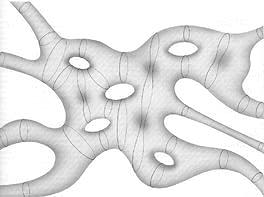WORLD SHEET is swept out by stringlike particles as they move and interact in spacetime. A stringlike particle, like an ordinary string, has extension in only one dimension. In superstring theory the strings are exceedingly small and move through a 10-dimensionai analogue of space and time. In the diagram time is the horizontal axis; closed strings, or loops (color), enter from the left and leave on the right. The closed strings sweep out world sheets that are deformed cylinders, that is, they are topologically equivalent to a cylinder. The corresponding diagrams for processes involving open strings are somewhat more complicated because the surfaces have boundaries traced out by the endpoints of the string. When two strings collide, they join to form a third string: two cylinders form a third cylinder. When strings split apart and rejoin, a hole is left in the world sheet. In quantum calculations all possible splittings and joinings between an initial state of strings and a final state must be considered. The topological structure of a world sheet describing these quantum-mechanical interactions is like that of a doughnut with an arbitrary number of holes. Properties of superstring theory for such world sheets are under intensive investigation and are closely related to several important topics in modern mathematics.

Roughly speaking, the strings served to bind together the quarks that make up the proton, the neutron and other hadrons. The original dual-resonance model could account only for particles, such as the pi meson, whose spin is an inte;ger in fundamental units. Such particles are called bosons, and in quantum mechanics they are sharply distinguished from the fermions, such as the electron and the proton, whose spin is one-half times an odd integer. In 1971 a variant of the original theory that included fermions was developed by Pierre M. Ramond of the University of Florida, Andr6 Neveu of the Ecole Normale Superieure in Paris and John H. Schwarz of the California Institute of Technology. The variant, known as the spinning-string theory, was the precursor of supersymmetric theories. Unfortunately both early string theories turned out to have several features that were considered serious liabilities at the time. First, the quantum-mechanical behavior of the original string theory for bosons makes sense only if spacetime has 26 dimensions! For the spinning-string theory and indeed for the current superstring theories the corresponding number of dimensions is 10. Furthermore, the theories were plagued by the fact that the states of lowest energy of the string must be tachyons, or particles that travel faster than light. A relativistic quantum theory with tachyons is inconsistent. Finally, the theories required the existence of massless spin-1 and spin-2 particles, which did not correspond to observed hadrons. Their properties were more like those of the photon, the graviton and the so-called weak gauge bosons that carry the weak force. Although Jo@l Scherk, an exceptional physicist who died at a tragically young age, and Schwarz suggested string theory might be reinterpreted as a theory of gravity and the other forces, the inconsistencies lurking in such a theory seemed overwhelming.

In the early 1970's there was a great resurgence of interest in quantum field theories based on point particles. As recently as 20 years ago the only successful quantum field theory was the quantum theory of electromagnetism I mentioned above, known as quantum electrodynamics, or QED. Yet only a few years later two more highly successful quantum field theories had been developed: the electroweak theory, which gives a unified description for both electromagnetism and the weak interaction, and quantum chromodynamics, or QCD, which describes how quarks bind together to form hadrons. These theoretical achievements were confirmed by remarkable experimental discoveries. In all such theories the role of symmetry is paramount. The idea of symmetry in the laws of physics is expressed by a set of transformations that form a mathematical structure called a group. For example, the physical laws governing the behavior of an apparatus do not depend on its orientation in space; the laws are said to be symmetric under rotations about any of the three independent spatial axes. All such rotations belong to the threedimensional rotation group designated 0(3); since rotations are specified by continuous angles, the group is a continuous symmetry group.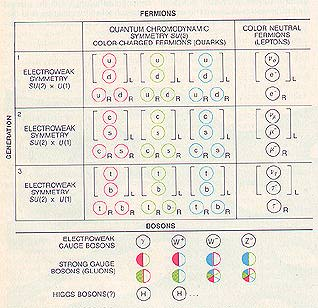STANDARD MODEL of elementary particles combines quantum chromodynamics (QCD), which is the theory of the "color," or strong, force, and the electroweak theory. In superstring theory all these particles should arise as massless states of a string vibration. The fermions are grouped in the table into three "generations" of particles. The grouping displays the symmetry of the underlying theory much as the grouping of chemical elements in Mendeleev's periodic table displays the symmetry underlying atomic physics. Fermions include the quarks, which carry one of three color charges associated with the symmetry group SU(3) of QCD, and the leptons, which carry no color charge. The color charges are represented here as red, green and blue. Quarks also carry electroweak "flavor," which is associated with the symmetry group SU(2) X U(1); six flavors are known and are indicated by the letters u, d, s, c, b and t. Leptons are subject only to the electroweak force. The leptons include the electron neutrino (ve), the electron (e-), the muon neutrino (vmu), the muon (mu-), the tau neutrino (vtau) and the tau (tau-). Gauge bosons transmit the forces. The electroweak gauge bosons include the photon (gamma) and the three massive particles W-, W+ and Zo. Eight strong gauge bosons, or gluons, carry color charges and anticolor charges, shown as solid color or as a color outline. There may also be Higgs bosons, which are responsible for the nonzero masses of particles. The subscripts L and R (for left and right) indicate handedness. For every particle except the neutrinos there is an antiparticle of the opposite handedness. The left-right asymmetry, most notable in the absence of right-handed neutrinos, is a signal that the weak interaction distinguishes among particles on the basis of handedness.

It turns out that for every continuous symmetry in physics there is a conserved quantity or charge. The continuous symmetry of rotations in space gives rise to the conservation of angular momentum. Many symmetries in particle physics are not related to ordinary space; instead they can be thought of as symmetries related to some so-called internal space. For example, in Maxwell's electromagnetic theory the internal space is regarded as a circle. Physical phenomena are independent of rotations about the circle just as they are independent of rotations in space. The conserved quantity associated with the symmetry is the electric charge.

The richest and most interesting field theories are the ones in which there can be independent symmetry transformations at each point in space and time. Such symmetries are called gauge symmetries, and the theories are called gauge theories. The earliest and simplest example of a gauge theory is again Maxwell's theory of electromagnetism. A circle is associated with each point in spacetime, and the laws of electromagnetism are not altered by independent rotations about each of the circles in this infinite set. This gauge symmetry is expressed by a symmetry group designated U(1). In any gauge theory there is a set of gauge particles that transmit the force between particles bearing a charge. In electrodynamics the gauge particle is a massless spinI particle called the photon. More general gauge theories with greater internal symmetry, such as the electroweak theory and QCD, also include massless spin-1 gauge particles. Such theories are known generically as Yang-Mills theories, after C. N. Yang of the State University of New York at Stony Brook and Robert L. Mills of Ohio State University. The forces described by Yang-Mills theories are nongravitational; they are called Yang-Mills forces. Finally, even Einstein's theory of gravity is a kind of gauge theory, but the spin of its gauge particle, the graviton, is 2.

One important aspect of a symmetry is that it can appear to be broken; the spontaneous appearance of a broken symmetry signals a change of state called a phase transition of a system of particles. For example, a lump of iron is not magnetized at high temperatures because the magnetic moments of the atoms point randomly in all directions. Since no direction is picked out, the system has rotational symmetry. When the iron is cooled, there is a change of phase: the atomic moments line up and the iron becomes magnetized in a particular direction.

The rotational symmetry appears to be broken. Note that the rotational symmetry of the laws governing the forces on a microscopic scale remains intact; the appearance of a broken symmetry is characteristic of a system (the lump of iron) made up of a large number of particles. Similarly, many theories of elementary particles, including superstring theories, require large gauge symmetry groups in order to give a unified account of diverse phenomena. Typically such large symmetry is apparent only at extremely high temperatures; the symmetry must appear to be broken at ordinary terrestrial temperatures if the theory is to be consistent with observations. For example, the electroweak theory describes a unified version of the electromagnetic and weak forces called the electroweak force, whose gauge symmetry is based on a group called SU(2) X U(1); the group is an extension of the group U(1) associated with electromagnetism. At ordinary temperatures, however, one observes two forces, the electromagnetic and weak forces, which are entirely distinct. The symmetry associated with the unification of the two forces becomes apparent only at temperatures much higher than 10^15 degrees Celsius. The success of the quantum field theories of point particles gave a new lease on life to quantum field theory, and many physicists turned their attention to more ambitious schemes for unification. Such schemes were almost invariably based not on string theories but on more elaborate, so-called grand-unified symmetries built into quantum field theories with point particles. The grand-unified schemes, which ignore gravity, were associated with symmetry groups called SU(5), SO(1O) or E6. These large symmetries can break into smaller symmetries associated with the group SU(3) of QCD and the group SU(2) X U(1) of the electroweak theory. The temperatures at which grandunified symmetries-and indeed the effects of quantum gravity-might become important are extraordinary: between 10^30 and 10^32 degrees C.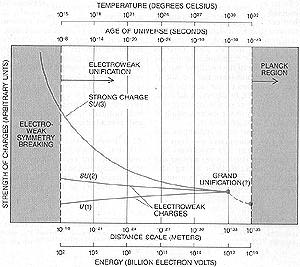CHARGES associated with the electroweak force and the strong force depend on the separation between the particles carrying the charges. Quantum theory leads to fluctuations in energy, which are manifest as a sea of "virtual" particles throughout space. The virtual particles carry charges that can shield the separated particles, thereby causing the variation in the strength of the charges. The other three horizontal coordinates indicate equivalent ways of understanding the variation. If one extrapolates from the measured values of the charges at an energy of 100 GeV (100 billion electron volts), the three charges appear to be equal in strength at about 10^17 GeV. The extrapolation suggests a larger, "grand unified" symmetry prevails above that energy, in which the electroweak and strong forces become indistinguishable. The grand-unified energy, however, may not be distinct from the energy at the Planck scale (10^19 GeV), in which case the force of gravity cannot be neglected.

According to current thinking about the origins of the universe, these temperatures were realized only between 10^-43 and 10^-38 second after the big bang. In spite of the brevity of this period, its implications for the subsequent evolution of the universe have been profound. In this way it turns out that the physics of the incomparably small is a key to the understanding of phenomena on a cosmic scale. Many of the new grand-unified theories also incorporated supersymmetry, a symmetry that transforms bosons and fermions into one another and thereby unifies particles having integer and half-integer spin. In a supersyrumetric theory there are equal numbers of bosons and fermions for any given mass. Several recent attempts have been made to combine Einstein's theory of gravity with supersymmetry. The resulting so-called supergravity theories belong to a new kind of gauge theory in which the gauge particle responsible for svpersymmetry is called the gravitino; its spin is 3/2. The most popular supergravity theory for a time was formulated in 11 spacetime dimensions: the four ordinary spacetime dimensions and seven additional spatial ones.

It is ironic from the current theoretical standpoint that one of the most important early discoveries in string theory was made in the process of studying higher-dimensional supergravity theories. In 1976 Ferdinando Gliozzi of the University of Turin, Scherk and David A. Olive of the Imperial College of Science and Technology in London suggested that the spinning-string theory could be made supersymmetric. The implications of this argument, however, were largely unrecognized, and work on string theory was virtually abandoned. It then lay dormant until 1980, when Schwarz and I (together with contributions from Lars Brink of the Chalmers Institute of Technology in Göteborg) began constructing and investigating the properties of string theories having spacetime supersymmetry. In order to understand why a solution to the problems of reconciling gravity with quantum theory has proved so elusive one must consider the implications of Heisenberg's uncertainty principle at distance scales of less than about 10^-15 meter.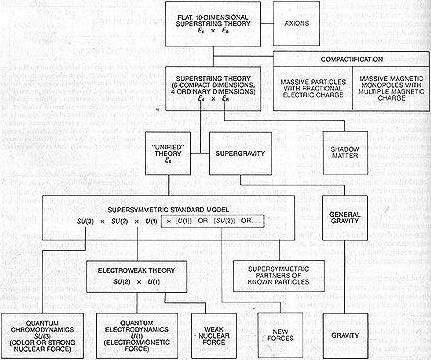SUPERSTRING THEORY that is based on the huge symmetry group E8 X E8 might make contact with observed physical phenomena by way of the connections shown in the logical diagram. When the effects of curvature in the six curled-up dimensions are considered, the theory resembles a supersymmetric grand-unified theory linked to supergravity, whose symmetry is associated with the group E6 X E8. That symmetry can break down to give the standard model of elementary particles and forces, which accounts for the three nongravitational forces. New particles and forces that may be required by certain interpretations of the theory are shown in color.

According to that principle, the more precisely a spatial measurement is made, the less precisely the momentum or the energy of the system being measured can be known. The uncertainties of energy are realized as fluctuations at short distances; because energy and mass are equivalent, the energy fluctuations can be manifest as the creation of "virtual" particles. Virtual particles and antiparticles can materialize out of the vacuum for a short time before they annihilate one another. The sea of virtual particles gives rise to multiparticle effects similar to the ones that arise for a substance such as a ferromagnet made up of many atoms. When the spatial resolution is less than about 10^-35 meter, the energy fluctuations become so huge that, ackcording to general relativity, virtual black holes form. The energy of the fluctuations, about 10^19 GeV (a GeV is one billion electron volts), is called the Planck energy, and the distance scale is called the Planck distance. Spacetime must therefore be considered highly curved on small scales; in a sense it is foamy. This conclusion has disastrous consequences for the usual computational techniques of quantum field theory because it invalidates the notion of spacetime as a smooth collection of points. In all such calculations one assumes the curvature of spacetime is small; if the assumption does not hold, the calculations give nonsensical infinite results. This argument suggests that at short distances or, equivalently, at high energies either general relativity or quantum mechanics (or both) must be revised. The corresponding argument cannot be made for strings, since superstring theory radically modifies the assumptions of general relativity at short distances. Indeed, as I outlined above, superstring theories may require a revision of general relativity at its most fundamental level: the idea of a curved spacetime, which is central to Einstein's theory, may have to be extended to the infinitely richer space of string configurations. The current understanding of the theory, however, begins with a more primitive model, in which the strings are treated as independent particles moving in some fixed spacetime background. Even with this restriction the quantum-mechanical treatment of a string leads to strong constraints on any superstring theory of gravity in higher dimensions. There are two kinds of strings, open and closed. Open strings have endpoints, and conserved charges, such as the electric charge, that are associated with the Yang-Mills forces are tied to the endpoints.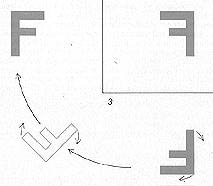CHIRALITY, or handedness, cannot be defined in an even number of spatial dimensions (or in odd-dimensional spacetime). For example, in two dimensions successive reflections of the asymmetric letter F through both coordinate axes can be undone by a rotation.

Particles associated with the vibrational states of an open string include the massless spin 1 gauge particles, but they do not include the graviton. When open strings collide, they can interact by touching and joining at their endpoints to form a third string; the third string can then split apart to form two final strings. Similarly, the two endpoints of an open string can join to form a closed string. The vibrational states of a closed string include the massless spin-2 graviton. Thus in any theory with open strings there are also closed strings, and in any string theory with closed strings it is inconsistent to neglect the force of gravity.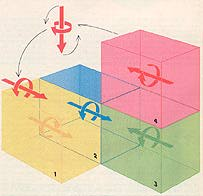MIRROR REFLECTION through all the coordinate axes in a space with an odd number of dimensions (or an even-dimensional spacetime) gives rise to the possibility of finding chiral particles in the space. For example, a neutrino (1) travels at the speed of light and spins in a left-handed sense about the direction of its motion. Its mirror image after reflection through all three axes (4) spins in a right-handed sense; in other words, it is not equivalent to any rotated version of the original neutrino. Only the left-handed neutrino occurs in nature, which shows the laws of physics are asymmetric with respect to handedness.

Accordingly, if the Yang-Mills forces, such as electromagnetism, are included in a string theory, they must be unified with gravity in an intimate way. A kind of theory in which the YangMills forces can be associated with closed strings was formulated by David J. Gross, Jeffrey A. Harvey, Emil J. Martinec and Ryan Rohm of Princeton University. Such a theory is known as heterotic, and it is the most promising kind of superstring theory developed so far. Its construction is quite strange. The charges of the Yang-Mills forces are included by smearing them out over the entire heterotic string. Waves can travel around any closed string in two directions, but on a heterotic closed string the waves traveling clockwise are waves of a 10-dimensional superstring theory; the waves traveling counterclockwise are waves of the original, 26-dimensional string theory. The extra 16 dimensions are interpreted as internal dimensions responsible for the symmetries of the Yang-Mills forces. As a string moves it sweeps out a two dimensional surface in spacetime called a world sheet, just as a point particle sweeps out a world line [see illustration on this page]. In classical, or non-quantum-mechanical, general relativity a particle moves along the world line that minimizes the so-called action of the particle: its energy as it moves through time. The action is proportional to the length of the world line, and so a path of least action is a geodesic, or the shortest distance between two points in spacetime. The motion of a string is treated in an analogous way. In a non-quantum mechanical approximation the string also moves in a way that minimizes its action. The action is proportional to the area swept out by the string, and so the world sheet must be a surface of minimum area. If time is regarded as a spatial dimension, the world sheet swept out by a closed string can be thought of as a kind of soap film that joins the string at its starting point and at the end of its path in spacetime. There is an enormous symmetry embodied in the condition that the motion of the string is determined by minimizing the area of its world sheet. The area is a geometric quantity that is in dependent of how the points on the two-dimensional sheet are labeled. No amount of distortion of the labeling can change the underlying geometry of the sheet, and physics is said to be symmetric under arbitrary relabelings of the world-sheet coordinates. Because of this symmetry, there is no physical significance to distortions of the world sheet that lie in the two di rections tangent to the surface of the sheet at any point. The only meaningful vibrations of the string are undulations of the world sheet perpendicular to its surface. Hence if the string is moving in d dimensions, two directions of vibration have no physical reality. All real vibrations are transverse vibrations in d-2 dimensions. It is worth noting that Such a constraint on the vibrational modes of photons, or waves in an electromagnetic field, is a hallmark of Maxwell's theory. There is no physical meaning to vibrations of the photon in the time direction, and longitudinal vibrations, which take place in the same direction as the wave is moving, are not possible for a wave traveling at the speed of light.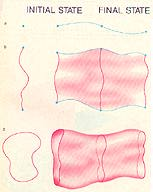LEAST-ACTION PRINCIPLE determines the classical, or non-quantum-mechanical, trajectory of a particle: if time is treated as a fourth spatial dimension,' the trajectory is the line of minimum length in spacetime joining the initial state and the final one (top). Similarly, according to the least-action principle, a string sweeps out a world sheet of minimum area in spacetime. Cross sections are shown at the left. Open strings carry charges at their endpoints (blue) that define the boundaries of the sheet (middle). Closed strings are loops, and so they have no endpoints (bottom). A string satisfying the least-action principle can vibrate in any combination of harmonic frequencies. Because deformations of the world sheet in the two directions tangential to its surface at any point are not distinct configurations of the sheet, only the vibrations perpendicular to its surface have physical reality.

The gauge symmetry of electromagnetism ensures the absence of such nonphysical vibrations. From this point of view one might expect insurmountable problems in a string theory, since a string is made up of an infinite number of points. Each point along the string vibrates, and so the potential for nonphysical vibrations of a string is infinitely greater than it is for a point particle such as the photon. The absence of such vibrations is guaranteed by the symmetry under coordinate relabelings. When the quantum mechanics of the string is considered, the same symmetry also introduces powerful constraints on possible string theories. It is in this sense that superstring theory is immensely elegant.

Up to now I have discussed the string as a classical particle. In quantum mechanics the motion of a particle is not precisely defined. When it moves through space, any particle tries, in effect, to take all possible paths between its initial state and its final state. The probability of each path is weighted so that a higher probability is assigned to paths of lower action; the classical path of least action is the most probable one. This formulation of the quantum behavior of a particle is called the method of summing over histories, and it was devised by Richard P. Feynman of Caltech. Its application to string theory has been most completely developed by Stanley Mandelstam of the University of California at Berkeley and by Alexander M. Polyakov of the Landau Institute for Theoretical Physics near Moscow. In superstring theory summing over histories requires summing over all the possible surfaces that join the initial and final states of a string or a set of interacting strings. The different paths can be thought of as fluctuations of the world sheet, which are just like the random, shimmering motions of a soap film at any temperature above absolute zero [see illustration above]. An important quantum-mechanical constraint on string theories was formulated in 1972 by Richard Brower of Boston University, Peter Goddard of the University of Cambridge and Charles B. Thorn of the University of Florida at Gainesville: Requiring symmetry under relabelings of the worldsheet coordinates of a free string undergoing quantum fluctuations is sufficient to determine the number of dimensions of the background spacetime in which the string is moving. Remember that in the original dual-resonance theory the number of spacetime dimensions is 26; in superstring theory 10 dimensions are required. This result had been intimated a year earlier in work by Claud W. V. Lovelace of Rutgers University. The constraint is a striking instance of how the formulation of the theory in terms of the twodimensional world sheet leads to a rich and highly determined structure for the space in which the string is moving.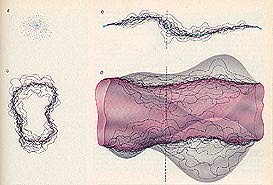"SUMMING OVER HISTORIES" is one way to take account of the probabilistic interpretation of the path of a particle in quantum mechanics. A point particle is pictured as moving simultaneously along all possible world lines in spacetime; a weight, or statistical probability, is assigned to each path in such a way that the shortest paths in spacetime are by far the most probable. The result is a collection of world lines that are densest along the classical trajectory (upper right). The cross section of the world lines through time, Indicated by the broken vertical line, is shown at the upper left. The density of the world lines through an arbitrary region of the cross section represents the probability of finding the string in that region at the corresponding time. Similarly, the quantum mechanics of a string is obtained by considering all possible world sheets with the same initial state and final state, weighted in such a way that the ones of smallest area are by far the most probable (lower right). The density of the closed loops in a given region of the temporal cross section of the world sheet (lower left) represents the probability of finding the complete string in that region at the corresponding time.

The vibrational frequencies of a superstring are determined by its tension, which is measured in units of energy per unit length, or mass squared in fundamental units. Since the theory is to describe gravity, the string tension must be closely related to the Planck energy, which is the only dimensional parameter in a gravitational theory. The tension of the string must therefore be on the order of 10^19 GeV squared; in more familiar units that is equal to a force of 10^39 tons. The frequencies of the normal vibrational modes of the string are therefore separated by huge gaps: particles corresponding to the lowest vibrational state are massless, but particles corresponding to the next vibrational state have a mass roughly equal to the mass of a speck of dust, which is enormous for an elementary particle. For higherfrequency vibrations the corresponding masses increase without limit. The significance of the massless states of superstring theory is that they include not only the graviton, the spin1 gauge particles of other forces and the spin-0 and spin-1/2 particles, but also the gravitino, the spin-3/2 gauge particle associated with supergravityThus for energies below the Planck energy the massless particles of superstring theories are the same ones found in supergravity theories.

A quantum string theory is different from the quantum theory of, a point particle in another important respect. Again consider the sum over histories of a single closed string: the sum includes all possible connected surfaces that can be stretched, twisted or otherwise smoothly deformed into a cylinder without tearing. All such surfaces are said to be topologically equivalent to the cylinder, and they include surfaces with long tentacles [see top illustration on page 55]. Under certain assignments of the time coordinate on the world sheet, the tentacles can be interpreted as motions in which two closed strings join to form a new one or in which a new closed string breaks away from the original one and disappears into the vacuum. Thus the string automatically interacts with the background space in which it moves, even though interactions are not explicitly included in the sum over histories. In contrast, the sum over histories of a single point particle does not include any information about interactions with the background space. Because of the nature of the interaction of a string with its background, even the motion of a single string can be consistently described only if the curvature of the background space is severely constrained. This result was originally implied by the work of Daniel Friedan of the University of Chicago, done in 1979. To appreciate the significance of the finding one must understand that the 10 dimensions required in a consistent superstring theory were not initially assumed to be curved at all. The theory was first formulated under the simplifying assumption that all 10 dimensions are equivalent, that is, all of them are "flat." If any superstring theory is to make sense of physical observations, however, six spatial dimensions must be highly curved. If the sum over the histories of the world sheet is to be consistent, the six dimensions must be curled up in one of a few special ways. They are said to form a kind of space called a Calabi-Yau space (after Eugenio Calabi of the University of Pennsylvania and Shing-Tung Yau of the University of California at San Diego); they may also form a generalnation of such a space called an orbifold. These spaces lead to a promising scheme for explaining the physics of the four observable dimensions.

Much of the interest in superstring theories follows from the rich structure that results by requiring the theory to be consistent. If the theory is to give a realistic quantum account of the Yang-Mills forces, there is an empirical constraint in addition to the re. quirement that there be only four observable dimensions: the theory must lead to the observed chirality, or handedness, of the weak force. The weak force is responsible for radioactive decay such as beta decay, which is an important reaction in the sun. The force is chiral (from the Greek word cheir, meaning hand) in the sense that it gives rise to effects whose mirror-reflected counterparts do not exist in nature. Unfortunately the quantum-mechanical version of any chiral gauge theory is likely to violate conservation laws such as the conservation of electric charge. Such a ,iolation is called a chiral anomaly; it signals a breakdown of gauge symmetry, which renders the theory inconsistent. Devising a theory that is chiral and yet avoids chiral anomalies is a delicate matter in four dimensions, and until recently it was thought to be impossible in 10 dimensions. it is only when space has an odd number of dimensions (or when spacetime has an even number) that the concept of chirality can be defined at all.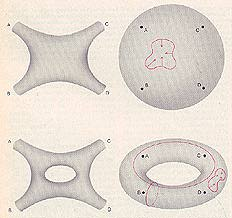INTERACTIONS AMONG STRINGS are represented by world sheets topologically equivalent to a sphere or to a doughnut with an arbitrary number of holes. The surfaces are continuous except at the points that represent the incoming or outgoing strings (A, B, C, D). The sphere is distinct in that any closed curve on its surface can be continuously shrunk to a point. Two kinds of closed curves on the torus cannot be continuously shrunk to a point.

In any number of dimensions chirality depends on the outcome of successive mirror reflections along all the spatial axes. When space has an odd number of dimensions, mirror reflection along each spatial axis gives an odd number of reflections, and so a left-handed shape is transformed into a right-handed one. When space has an even number of dimensions, a reflection along each spatial axis leaves any shape unaltered: the reflected image can be rotated into the original shape. For example, the popular 1 I-dimensional theory of supergravity cannot lead to a chiral theory because it is formulated in 10 spatial dimensions (an even number). With nine spatial dimensions superstring theory can be chiral. Even when the higher-dimensional theory is chiral, the process of curling up the extra dimensions generally washes out chirality. It is now thought that the observed chirality can be explained only if the chiral higherdimensional theory is initially formulated to include the gauge symmetry group of a Yang-Mills force, in addition to the gravitational force. The symmetry group must be present in the flat, 10-dimensional theory before the possibility that the extra dimensions curl up can be considered. In August, 1984, to the surprise of many physicists, Schwarz and I showed that a chiral theory free of anomalies can be formulated in 10 dimensions, provided the symmetry group of the Yang-Mills force is one of two special groups. The groups must be either SO(32), a generalization to 32 internal dimensions of the symmetry of spacetime, or the group E8 x E8, the product of two exceptional, continuous groups that were first discovered by the French mathematician Elie Cartan. A third group known as 0(16) X 0(16), which also leads to freedom from anomalies, has recently been noted; its symmetry is a subsymmetry of the other two possible groups. The fact that the quantum consistency of a theory including gravity leads to an almost unique prediction of the unifying symmetry group was an exciting development. It has led to the current wave of enthusiasm for superstring theory.

The quantum mechanics of a single string is only an approximation to a full theory, not yet developed, in which the interactions of arbitrary numbers of strings would be described. Interactions arise when strings join or split. The probability that a given set of incoming strings leads to a given set of outgoing strings is determined by an infinite sequence of so-called Feynman diagrams. The diagrams describe all possible joinings and splittings of the world sheets, summed over histories for each case. The simplicity of the sequence of possible Feynman diagrams for strings contrasts sharply with the complexity of the possible Feynman diagrams for point particles. Consider the possible diagrams for two interacting closed strings. First the two strings can join and then split apart. The Feynman diagram for the process is topologically equivalent to the surface of a sphere [see illustration on opposite page]. In the next term in the sequence the intermediate string splits into two strings, which then rejoin. The diagram is topologically equivalent to a torus, or the surface of a doughnut. The sequence of diagrams is continued simply by adding holes to the doughnuts: two holes, three holes and so on. Requiring symmetry under relabelings of coordinates on the torus introduces strong, new constraints to superstring theory. If the torus is cut in either one of two ways, twisted and glued back together, its topology is unchanged; the twisted coordinate system, however, cannot be continuously returned to the original one without cutting the surface again [see illustration on next page]. The invariance of the torus under such changes in coordinates leads to an important constraint in the heterotic superstring theory. A heterotic string can move through flat, 10-dimensional spacetime only if the symmetry of the nongravitational forces in the theory is described either by the group F-8 X E8, the group spin (32)/Z2, a variant of SO(32), or the group O(16) X O(16). The restriction to these groups is striking. Remember that Schwarz and I had earlier singled out the same groups by requiring the absence of chiral anomalies in any consistent 10-dimensional chiral gauge theory. The constraint that leads to these groups in the heterotic theory is related to remarkable mathematical properties of certain 16-dimensional lattices of points. Such lattices are constructed from the 16 internal dimensions I mentioned above. There is another and almost equally striking feature of the torus diagram that indicates the profound difference between string theories and the theories of point particles. Among the many kinds of one-loop Feynman diagrams for point particles that are the analogues of the torus diagram, there are diagrams that give infinite answers when the sum over histories is calculated. Such infinities arise because the diagrams for point particles include the sum over histories when two interaction points are arbitrarily close in spacetime. The contributions to the entire process of each one of an indefinitely large number of fluctuating paths must be taken into account, and that leads to infinite answers.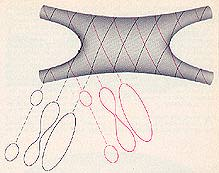PRECISE INSTANT at which two strings join cannot be objectively specified because the definition of the time coordinate of a point on the world sheet depends on the observer. The colored coordinate lines indicate contours of equal time as defined by one observer. To this observer the two incoming strings appear to join at the colored point. To another observer the black lines are contours of equal time; the strings appear to join at the black point.

In contrast, on a string Feynman diagram no one point can be identified as a splitting or joining point of two strings [see illustration on preceding page]. Hence, at least in the one-loop diagram of string interactions, the concept of an interaction point does not arise. The sum over histories leads to finite answers in precisely those superstring theories whose Yang-Mills forces are associated with the symmetry groups E8 X E8 or SO(32).

An unfortunate feature of any quantum theory of gravity, whose natural energy scale is necessarily enormous, is the difficulty of extracting testable or observable predictions at more modest energies. For example, although superstring theory is initial ly formulated in 10 flat spacetime dimensions with huge unifying symme tries, it is only at an inconceivably small distance scale or, equivalently, at extremely high energy or temperature that the curvature of the extra six dimensions might be negligible and the full symmetry of the theory would be realized. Thus any attempt to derive low-energy consequences of the the ory must be considered speculative, and there are severe problem@ in ob taining concrete predictions. Never theless, it is now possible to give a plausible picture of how superstring theory might make contact with the phenomena observed in accelerators. Many aspects of this picture are based on topological arguments, whose application to physics has been pioneered by Edward Witten of Princeton. Witten noted that the curling up of the extra dimensions and symmetry breaking go hand in hand: there must be a strong correlation between the curvature of the extra dim6nsions and the way in which the huge symmetry associated with the Yang-Mills forces breaks down to a smaller symmetry. In flat IO-dimensional spacetime the average values of the fields associated with the E8 X E8 or SO(32) Yang-Mills forces are zero. Witten showed that if the curvature of the space is nonzero in certain dimensions, the average values of these fields in the curved space must be nonzero in the same dimensions. A nonzero average value for a field signals a transition to a phase with less symmetry, just as the nonzero magnetization of a ferromagnet signals the transition to the less symmetric magnetized phase. Thus if six dimensions of the original flat spacetime become highly curved, the Yang-Mills forces in the theory of the resulting curved space are unified by a subsymmetry of E8 X E8 or SO(32). This result is just what one would want. So far it has not been proved that the six spatial dimensions must be curled up in superstring theory. As I stated above, however, the requirement that the theory retain its large symmetry under relabelings of the coordinates of the world sheet forces the curledup dimensions to form a Calabi-Yau space or perhaps an orbifold. Assuming six dimensions are curled up in this way, Philip Candelas of the University of Texas at Austin, Gary T. Horowitz of the University of California at Santa Barbara, Andrew E. Strominger of the Institute for Advanced Study in Princeton and Witten have devised an imaginative scheme for understanding how superstring theory might relate to phenomena measured at the energies of present experiments.

In the scheme of Candelas and his coworkers the average value of the electric field associated with the group E8 x E8 is set equal to the nonzero value of the curvature of the six-dimensional space. Because of special features of Calabi-Yau spaces (or the associated orbifolds), the symmetry breaks down in a special way to the group E8 x E8, while still preserving its supersymmetry. It is reassuring to derive the group E6 in this way because it is one of the groups studied in efforts to unify the Yang-Mills forces. The extra group factor E8 enlarges the symmetry enormously, but it do not directly affect the observed particles.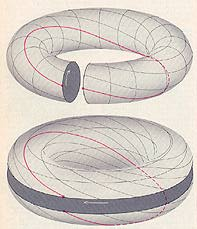INVARIANCE of the, labeling of the space and time coordinates on a world sheet with the topology of the torus is an even stronger requirement than it is on the sphere. A torus can be cut like a sausage (upper diagram) or like a yo-yo (lower diagram). One side of the cut can be given a full twist with respect to the other side, and the two boundaries can then be "glued" back together. The points on the surface are thereby labeled according to a set of coordinates (black and colored curves) that cannot be continuously deformed to the original set of coordinates. The requirement that superstring theory not be sensitive to such relabelings leads to strong conditions on the symmetry groups associated with the nongravitational forces of the theory. Symmetry groups must be either SO(32), E8 X E8 or 0(16) X 0(16).

Particles carrying the forces assciated with the extra E8 symmetry are neutral with respect to the observed Yang-Mills forces, but they should exert gravitational attraction. It has been speculated that matter made up such particles, which has been called shadow matter, might account for part of the unseen mass known to be present in the universe. One immediate prediction of the interpretation of superstring theory the existence of a particle called the axion. Such a particle has been postulated in order to avoid a violation of important symmetry in QCD, the theory of the strong interaction. Although the axion has not yet been found, several groups of investigators are planning experiments that could detect it. Many other physical consequences follow from purely topological properties of the six curled-up dimensions. For example, one of the most basic topological properties of a space is the Euler number. If the curled-up space were two-dimensional, the Euler number would be 2 minus twice the genus of the surface, where the genus is the number of holes. In six dimensions the Euler number is less easy to describe. The observed fermions are naturally grouped into "generations" of quarks and leptons [see illustration, on page 46 (The leptons are fermions that do not strongly interact.) Three generations have been discovered so far, and the is probably at most one more. The predicted number of generations is half the Euler number of the six-dimensional compact space.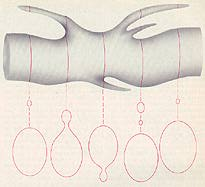QUANTUM-MECHANICAL ANALYSIS of a single world sheet leads to strong constraints on the kind of curled-up background space in which the string is moving. The quantum-mechanical sum over histories includes configurations of the world sheet that describe strings materializing from the vacuum (left) or breaking away and disappearing into the vacuum (right). Thus string interactions with the background are automatically included in the quantum treatment of one string. The only background spaces known to be consistent with such effects are the so-called Calabi-Yau spaces or related spaces called orbifolds.

Because only few of the known Calabi-Yau spaces and orbifold spaces have a small Euler number, only a few such spaces are candidates for describing observing physical phenomena. The six-dimensional spaces known to have a small Euler number are spaces that also have "holes" in them [see illustration on next page]. The presence of the holes allows the fields associated with the symmetry group E6 be trapped. The trapping of the field causes the symmetry to break down further, without the need for the massive so-called Higgs particles usually associated with this symmetry breaking. Quantum chromodynamics and the electroweak theory of standard elementary-particle physics, whose sy metries are described by the group product SU(3) X SU(2) X U(1), can readily be obtained from this latter symmetry breaking. It is likely th the same symmetry breaking will also give rise to additional forces associated with remnants of E6. There are many ways a closed string, can get trapped in the curled-up space. For example, the string can wind through a hole; its vibrations might then be manifest as massive particles with fractional electric charge or massive magnetic monopoles with multiple magnetic charges.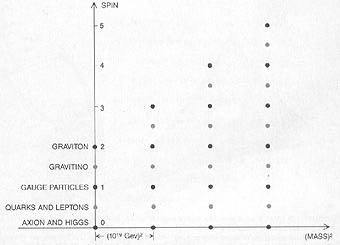SPECTRUM OF STRING STATES is plotted for the heterotic string theory in which the extra six dimensions of spacetime have been curled up. Each black dot represents a set of bosons and each colored dot represents a set of fermions. All string states that correspond to known particles are massless states; the states with nonzero mass form an infinite series whose masses are a whole number times the square of the Planck mass, which is 10^11 GeV. For each mass the number of fermion states is equal to the number of boson states. If each possible spin direction is counted as a different state, there are 8,064 massless states, and 18,883,584 states at the first mass level; the number increases exponentially thereafter.

Alhough superstring theory has already opened many lines of investigation in both physics and mathematics, there are fundamental questions that cannot be answered until more is understood about the structure of the theory. For example, why is observed spacetime approximately flat and four-dimensional? Can one prove that six dimensions are curled up? More to the point, can one explain why the four familiar dimensions are so large? Just after the big bang, when the size of the observed universe was on the order of the Planck distance, 10^-35 meter, all 10 dimensions must have been curled up. During the subsequent expansion of the universe all but six of the dimensions must have begun to unfold and expand. A consistent account of the process might lead to the prediction of an observable cosmic remnant. These issues are closely related to another fundamental question: Why is the cosmological constant so close to zero? This constant describes the part of the curvature of the universe that is not caused by matter; its value has been determined to be zero to within one part in 10^120, which makes it the most accurate measurement in all science. If superstring theory can account for the value, the explanation would be a convincing test of the theory. As I mentioned above, the natural mass scale of superstring theory is so large that the masses of the heaviest particles observable in current acceler-' ators are infinitesimal by comparison. As long as the supersymmetry included in superstring theories remains unbroken, the masses of the particles we observe are zero. The small, nonzero values of particle masses are therefore correlated with the breaking of supersymmetry at the relatively low temperatures of the world we observe. Nevertheless, there are strong arguments that the breaking of supersymmetry, and hence the precise values for the masses of particles observed in highenergy accelerators, cannot be understood in superstring theory as it is currently formulated. In order to resolve such issues it is presumably necessary to understand the deep principles on which the theory is based. In a sense the development of superstring theories is in sharp contrast to the development of general relativity. In general relativity the detailed structure of the theory follows from Einstein's penetrating insights into the logic of the laws of physics. In superstring theories certain details have come first; we are still groping for a unifying insight into the logic of the theory. For example, the occurrence of the massless graviton and gauge particles that emerge from superstring theories appears accidental and somewhat mysterious; one would like them to emerge naturally in a theory after the unifying principles are well established.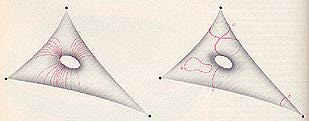ORBIFOLD SPACE may form the highly curved, six-dimensional background space in which strings move. The diagrams give an impressionistic rendering of a two-dimensional version of an orbifold. A closed, two-dimensional surface has been stretched out to form three sharp points, which are called conical singularities. The surface is considered flat everywhere except at the singularities in the sense that it can be cut and laid out on a flat plane. Similarly, a sixdimensional orbifold is flat everywhere except at isolated conical singularities; there the curvature is infinite. The hole in the center of the surface in the diagram indicates that the orbifold is not "simply connected": it can be cut through without falling into separate pieces. A closed path looping through a hole in the six-dimensional orbifolds of interest can be unwound after a fixed number of loops. Such holes cause lines of electromagnetic potential associated with the symmetry group E6 to become trapped (colored curves at left). The entrapment causes E6 to break down to a smaller symmetry group, similar to the one associated with the standard model. A closed string moving in an orbifold (right) can move freely (a), but it can also become trapped in various ways. If it loops around a conical singularity, the curvature prevents it from unlooping (b). It can also loop through the hole (c) or wind first through the hole and then around a conical singularity (d). A string looping through the hole can lead to massive particles with fractional electric charge or to massive magnetic monopoles with multiple magnetic charges.

How can the logic of superstring theory be discovered? The principles of general relativity must be a special case of the more general principles of superstring theory, and so in a sense general relativity can serve as a guide. For example, by developing an analogy with general relativity I explained above how superstring theories are expected to extend the idea of ordinary spacetime to the space of all possible configurations of a string. This idea is under intensive investigation. Even more radical is the suggestion that the theory should be studied solely in its two-dimensional formulation; no reference at all would then be made to the coordinates of the space and time in which we live. Whatever the resolution of these possibilities may be, developing a deep understanding of the logical status of the theory will undoubtedly lead to profound mathematical and physical problems. It should also lead to a better understanding of the predictions of superstring theory. The prospect is for a period of intellectual ferment and rapid advance.